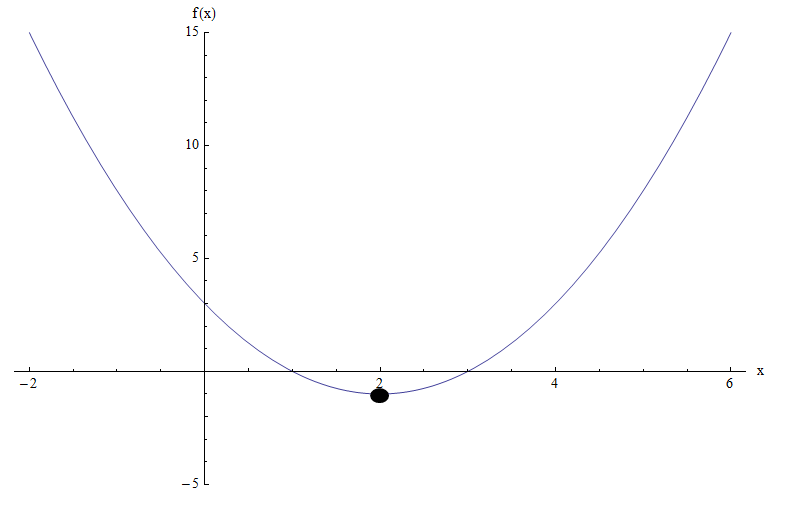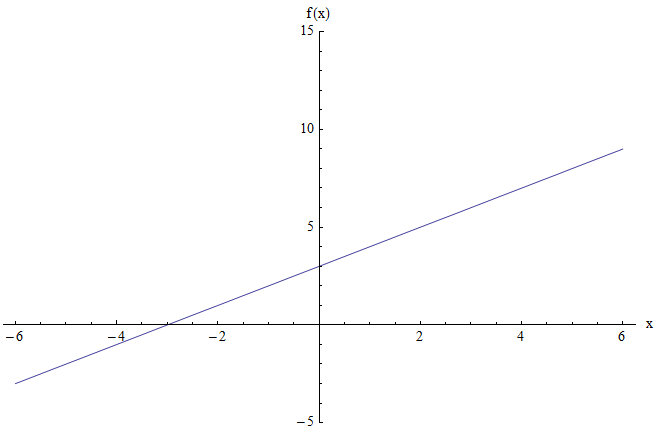# How to Find Range

Learning how to find the range of a function can prove to be very important in Algebra and Calculus, because it gives you the capability to assess what values are reached by a function. Or in other words, it allows you to find the set of all the images via the function

The task of finding what points can be reached by a function is a very useful one. For example, you may have a production function $$q(x)$$, which gives you the amount of output obtained for $$x$$ units of input.

We would like to know how many input units are needed to produce $$b$$ units of output. So in other words, we need to find $$x$$ so that $$q(x) = b$$, which is another way of asking whether or not $$b$$ is in the range of the function $$q(x)$$.In this tutorial we will concentrate more on the mechanics of finding the range. For a more conceptual approach to domain and range, you can check this tutorial .

## The Algebraic Way of Finding the Range of a Function

Same as for when we learned how to compute the domain, there is not one recipe to find the range, it really depends on the structure of the function $$f(x)$$.

Yet, there is one algebraic technique that will always be used. This is THE way you find the range. Pay attention:

Say that we need to get the range of a given function $$f(x)$$. Then, we will consider a generic real number $$y$$ and we will try to solve for $$x$$ the following equation:

$f(x) = y$

We need to determine for which values of $$y$$ the above equation can be solved for $$x$$. That is it. Of course, that could be hard to do, depending on the structure of the function $$f(x)$$, but its what you need to do.

So this is the algebraic way, the way how to find range of a function without graphing.

### EXAMPLE 1

Find the range of the function $$\displaystyle f(x) = \frac{x+1}{x-3}$$:

We proceed using the algebraic way: Let $$y$$ be a number and we will solve for $$x$$ in the following equation: $$f(x) = y$$. The value $$y$$ is in the range if $$f(x) = y$$ can be solved for $$x$$.

In this case we have:

$\large f(x) = y \Leftrightarrow \frac{x+1}{x-3} = y$ $\Rightarrow \,\,\,x+1 = y\left( x-3 \right)$ $\Rightarrow \,\,\,x+1 = yx-3y$ $\Rightarrow \,\,\,x-yx=-1-3y$ $\Rightarrow \,\,\,x\left( 1-y \right)=-1-3y$ $\Rightarrow \,\,\,x=\frac{3y+1}{y-1}$

Therefore, when will $$x$$ be well defined? Almost for all $$y$$, except for when $$y = 1$$, because in that case we have a division by $$0$$. Hence, the range of $$f$$ in this case is the whole real line, except for 1.

If we use interval notation, we can write $$Range(f) = (-\infty, 1) \cup (1, +\infty)$$.

### EXAMPLE 2

Find the range of the function $$f(x) = x^2 - 4x + 3$$:

Again, we proceed using the algebraic way, so you know the drill: Let $$y$$ be a number and we will solve for $$x$$ in the following equation: $$f(x) = y$$. The value $$y$$ is in the range if $$f(x) = y$$ can be solved for $$x$$.

In this case we have:

$\large f(x) = y \Leftrightarrow x^2 - 4x + 3 = y$ $\Rightarrow \,\,\, x^2 - 4x + 3 - y = 0 \text{ (This is a quadratic equation in x)}$ $\Rightarrow \displaystyle \,\,\, x = \frac{-b \pm \sqrt{b^2 - 4ac}}{2a}$ $\Rightarrow \displaystyle \,\,\, x = \frac{-(-4) \pm \sqrt{(-4)^2 - 4(1)(3-y)}}{2(1)}$ $\Rightarrow \displaystyle \,\,\, x = \frac{4 \pm \sqrt{16 - 4(3-y)}}{2}$ $\Rightarrow \displaystyle \,\,\, x = \frac{4 \pm \sqrt{16 - 12 + 4y}}{2}$ $\Rightarrow \displaystyle \,\,\, x = \frac{4 \pm \sqrt{4 + 4y}}{2}$ $\Rightarrow \displaystyle \,\,\, x = 2 \pm \sqrt{1+y}$

Now, seeing this final expression, when will $$x$$ be well defined? We need to have that the argument of the square root needs to be non-negative, so we need:

$1+y \ge 0$

which means that $$y \ge -1$$. If we use interval notation, we can write $$Range(f) = [-1, +\infty)$$.

In this example, we could have solved it using the fact that $$f(x) = x^2 - 4x + 3$$ is a quadratic function, and its graph is a parabola that opens upward.

The minimum point of this parabola is reached at the vertex. The x-coordinate of the vertex is:

$\displaystyle x_V = \frac{-b}{2a} =\frac{-(-4)}{2(1)} =\frac{4}{2} = 2$

Now, the y-coordinate of the vertex is simply found by plugging the value $$x_V = 2$$ into the quadratic function:

$y_V = f(x_V) = 2^2 - 4(2) + 3 = -1$

Since the minimum value reached by the parabola is $$-1$$, we conclude that the range is $$[-1, +\infty)$$, which is the same conclusion as the one found algebraically.

The graph of the function $$f(x) = x^2 - 4x + 3$$ makes it even more clear:We can see that, based on the graph, the minimum is reached at $$x = 2$$, which is exactly what was found to the x-coordinate of the vertex.

The risk of using the graph to find the range is that you could potentially misread the critical points in the graph and give an inaccurate evaluation of the where the function reaches its maximum or minimum.

## Other Strategies for Finding Range of a function

As we saw in the previous example, sometimes we can find the range of a function by just looking at its graph.

For example, say you want to find the range of the function $$f(x) = x + 3$$. The graph is shown below:The graph above does not show any minimum or maximum points. Moreover, when $$x$$ is large and positive, the value of the function is also large and positive. And analogously, when $$x$$ is very negative, the value of the function is also very negative.

The intuition is that function can take as negative and as positive as we want values, by selecting large enough (positive or negative) $$x$$ values. And then, the conclusion is that the range is the whole real line, which is $$(-\infty, +\infty)$$ using interval notation.

Such analysis is correct in terms of the result, but it is flimsy in terms of the reasoning. The "graphical method" to find the range has that problem: it is appealing from an intuitive point of view, but it is rather thin in terms of content.

Normally, if possible, we should prefer the analytical/algebraic way. In the example, we need to solve for $$x$$:

$x + 3 = y$ $\Rightarrow \,\, x = y - 3$

So, is there any restriction on $$y$$ for $$x$$ to be well defined? Not at all, so then, there is no restrictions on $$y$$ and the conclusion is that the range is the whole real line.

You can check this article you want to know how to find the domain of a function instead.

There are many good algebraic reasons for finding the range, one of them is because it is a part of the processes for finding the inverse of a function .